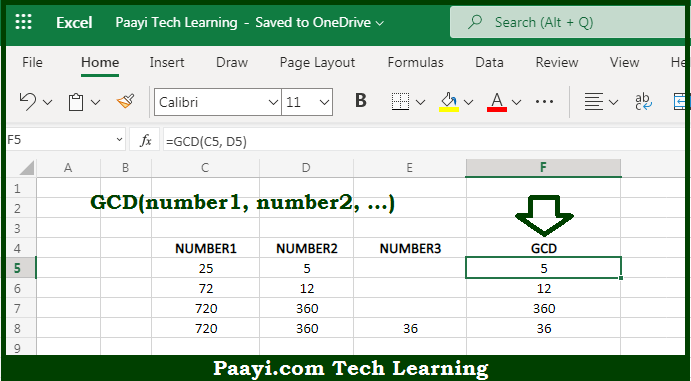# Learn How to Use Microsoft Excel GCD Function

Written by | 0 Comments | 590 Views

In this article, you will learn how to use the Microsoft Excel GCD function and its prime function in Microsoft Excel. You will also get to know the Microsoft Excel GCD function return value and syntax with the help of some examples.

Microsoft Excel GCD Function

The main function of the Microsoft Excel GCD function is to get the greatest common divisor of two or more numbers. So, with the help of the GCD function, you can able to return the greatest common divisor of two or more integers. It should be noted that the GCD is can be defined as the largest number that goes into all the given numbers, without leaving a remainder. So, with the help of the Microsoft Excel GCD function, you can easily get the greatest common divisor of two or more numbers.

Return Value of GCD Function

The return value will be the largest positive integer that divides the numbers with leaving a remainder.

Syntax of GCD Function

=GCD(number1, [number2], ...)

Where the arguments:

• number1: This is the first number.
• number2: This is the second number (optional).

How to Use Microsoft Excel GCD Function?So we know that Microsoft Excel GCD function you can able to get the greatest common divisor of two or more numbers. So, with the help of the GCD function, you can able to return the greatest common divisor of two or more integers. It should be noted that the GCD is can be defined as the largest number that goes into all the given numbers, without leaving a remainder. So, with the help of the Microsoft Excel GCD function, you can easily get the greatest common divisor of two or more numbers.